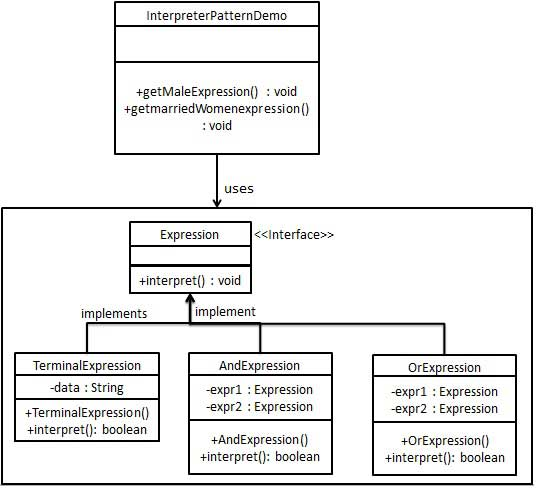# 解释器模式

## 实现

InterpreterPatternDemo，我们的演示类使用 Expression 类创建规则和演示表达式的解析。### 步骤 1

```public interface Expression {
public boolean interpret(String context);
}```

### 步骤 2

```public class TerminalExpression implements Expression {

private String data;

public TerminalExpression(String data){
this.data = data;
}

@Override
public boolean interpret(String context) {
if(context.contains(data)){
return true;
}
return false;
}
}```
```public class OrExpression implements Expression {

private Expression expr1 = null;
private Expression expr2 = null;

public OrExpression(Expression expr1, Expression expr2) {
this.expr1 = expr1;
this.expr2 = expr2;
}

@Override
public boolean interpret(String context) {
return expr1.interpret(context) || expr2.interpret(context);
}
}```
```public class AndExpression implements Expression {

private Expression expr1 = null;
private Expression expr2 = null;

public AndExpression(Expression expr1, Expression expr2) {
this.expr1 = expr1;
this.expr2 = expr2;
}

@Override
public boolean interpret(String context) {
return expr1.interpret(context) && expr2.interpret(context);
}
}```

### 步骤 3

InterpreterPatternDemo 使用 Expression 类来创建规则，并解析它们。

```public class InterpreterPatternDemo {

//规则：Robert 和 John 是男性
public static Expression getMaleExpression(){
Expression robert = new TerminalExpression("Robert");
Expression john = new TerminalExpression("John");
return new OrExpression(robert, john);
}

//规则：Julie 是一个已婚的女性
public static Expression getMarriedWomanExpression(){
Expression julie = new TerminalExpression("Julie");
Expression married = new TerminalExpression("Married");
return new AndExpression(julie, married);
}

public static void main(String[] args) {
Expression isMale = getMaleExpression();
Expression isMarriedWoman = getMarriedWomanExpression();

System.out.println("John is male? " + isMale.interpret("John"));
System.out.println("Julie is a married women? "
+ isMarriedWoman.interpret("Married Julie"));
}
}
```

### 步骤 4

```John is male? true
Julie is a married women? true```